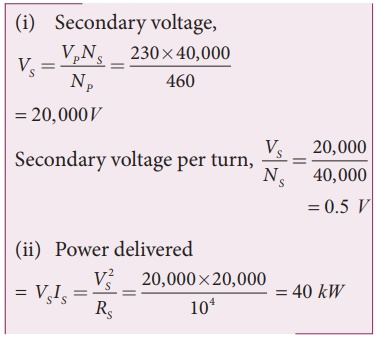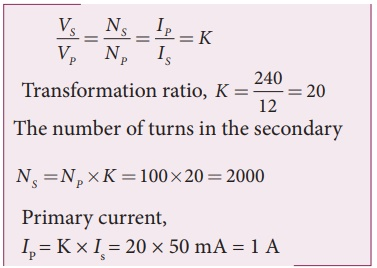Home | | Physics 12th Std | Advantages of AC in long distance power transmission

# Advantages of AC in long distance power transmission

Electric power is produced in a large scale at electric power stations with the help of AC generators.

Advantages of AC in long distance power transmission

Electric power is produced in a large scale at electric power stations with the help of AC generators. These power stations are classified based on the type of fuel used as thermal, hydro electric and nuclear power stations. Most of these stations are located at remote places. Hence          the electric power generated is transmitted over long distances through transmission lines to reach towns or cities where it is actually consumed. This process is called power transmission.

But there is a difficulty during power transmission. A sizable fraction of electric power is lost due to Joule heating (i 2 R) in the transmission lines which are hundreds of kilometer long. This power loss can be tackled either by reducing current i or by reducing resistance R of the transmission lines. The resistance R can be reduced with thick wires of copper or aluminium. But this increases the cost of production of transmission lines and other related expenses. So this way of reducing power loss is not economically viable.Since power produced is alternating in nature, there is a way out. The most important property of alternating voltage that it can be stepped up and stepped down by using transformers could be exploited in reducing current and thereby reducing power losses to a greater extent.At the transmitting point, the voltage is increased and the corresponding current is decreased by using step-up transformer (Figure 4.38). Then it is transmitted through transmission lines. This reduced current at high voltage reaches the destination without any appreciable loss. At the receiving point, the voltage is decreased and the current is increased to appropriate values by using step-down transformer and then it is given to consumers. Thus power transmission is done efficiently and economically.

Illustration:

An electric power of 2 MW is transmitted to a place through transmission lines of total resistance, say R = 40 Î©, at two different voltages. One is lower voltage (10 kV) and the other is higher (100 kV). Let us now calculate and compare power losses in these two cases.

Case (i):

P = 2 MW; R = 40 Î©; V = 10 kVCase (ii):

P = 2 MW; R = 40 Î©; V = 100 kVThus it is clear that when an electric power is transmitted at higher voltage, the power loss is reduced to a large extent.

EXAMPLE 4.16

An ideal transformer has 460 and 40,000 turns in the primary and secondary coils respectively. Find the voltage developed per turn of the secondary if the transformer is connected to a 230 V AC mains. The secondaryisgiventoaloadofresistance104â„¦. Calculate the power delivered to the load.

Solution

NP = 460 turns; NS = 40,000 turns

VP = 230 V; RS = 104 â„¦EXAMPLE 4.17

An inverter is common electrical device which we use in our homes. When there is no power in our house, inverter gives AC power to run a few electronic appliances like fan or light. An inverter has inbuilt step-up transformer which converts 12â€†V AC to 240 V AC. The primary coil has 100 turns and the inverter delivers 50 mA to the external circuit. Find the number of turns in the secondary and the primary current.

Solution

Vp = 12 V; Vs = 240 V

Is = 50mA; Np = 100 turnsStudy Material, Lecturing Notes, Assignment, Reference, Wiki description explanation, brief detail
12th Physics : Electromagnetic Induction and Alternating Current : Advantages of AC in long distance power transmission |